# Amazon DCF Valuation Model

Guide to Building a DCF Valuation Model of Amazon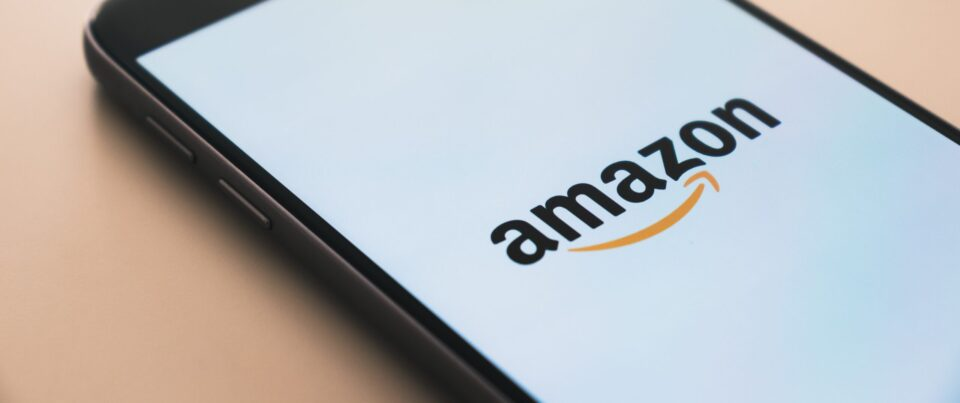## Amazon Valuation Model Introduction

The premise of the discounted cash flow (DCF) model states the value of a company is equal to the present value (PV) of all its expected future free cash flows (FCFs).

Formally, a DCF-derived valuation is referred to as the “intrinsic value”, in which the company’s fundamentals determine its approximate worth.

In order to perform a DCF valuation on a company – Amazon (NASDAQ: AMZN), in our case – the process consists of six primary steps:

1. Free Cash Flow Projection: The future FCFs expected to be generated by the company must be projected, with the standard DCF consisting of a two-stage model in which the explicit forecast period is a five-to-ten year time horizon.
2. Terminal Value (TV): Beyond a certain point, a company’s financials are no longer able to be projected within reason (i.e. the time horizon is too far out), so an approximation becomes necessary to capture the so-called “terminal value” of the company.
3. WACC Calculation: The FCFs must be discounted to the present date to account for the “time value of money” concept. If the FCFs are unlevered, the appropriate discount rate to use is the weighted average cost of capital (WACC), which represents the cash flows attributable to all capital providers, both debt and equity.
4. Enterprise Value Calculation: The sum of the discounted FCFs and the discounted terminal value equals the enterprise value (TEV) of the company.
5. Enterprise Value → Equity Value: To move from the enterprise value to the equity value, net debt and any non-equity claims must be subtracted.
6. DCF-Derived Share Price: As part of the final step, the implied share price can be calculated by dividing the company’s equity value by the number of diluted shares outstanding.
###### Model Disclaimer

Frankly, the most difficult step of the entire process is actually the first step, as creating a defensible forecast requires spending substantial studying the company, its financial statements, and the industry conditions.

The growth prospects of a company and how it’ll fare over the long run as new competitors emerge and new trends develop is not an easy task by any means.

For those reasons, it bears repeating that our model is meant solely for educational purposes.

Our objective here is to teach the mechanics of a 3-statement model and the steps to integrate the 3-statement model into a DCF model to arrive at the estimated intrinsic value.

So as we complete this modeling tutorial, keep in mind that our model’s assumptions are not supported by the necessary time spent researching Amazon’s financial statements and combing through all public filings.

## AMZN Business Description and Revenue Model

A general business description is provided in Amazon’s Form 10-K filing.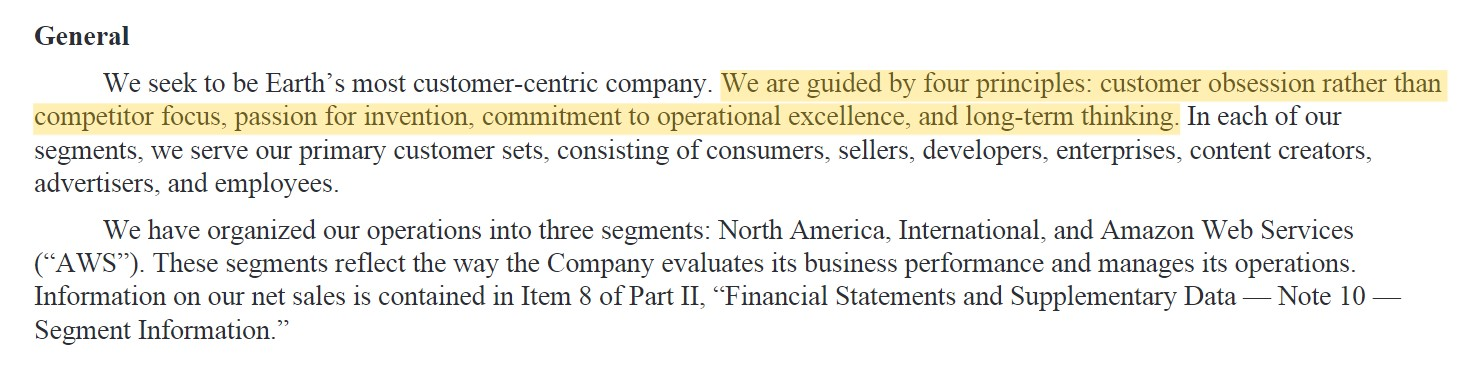Amazon separates its operations into three distinct segments for measuring business performance.

1. North America
2. International
3. Amazon Web Services (AWS)

Amazon is most widely recognized as a pioneer in e-commerce, a segment that benefited tremendously amid the COVID-19 pandemic.

In terms of revenue concentration, the primary source of Amazon’s revenue stems from their “North America” segment, which is comprised primarily of the unit sales of products/services to customers, sales by third-party sellers, and advertising revenue.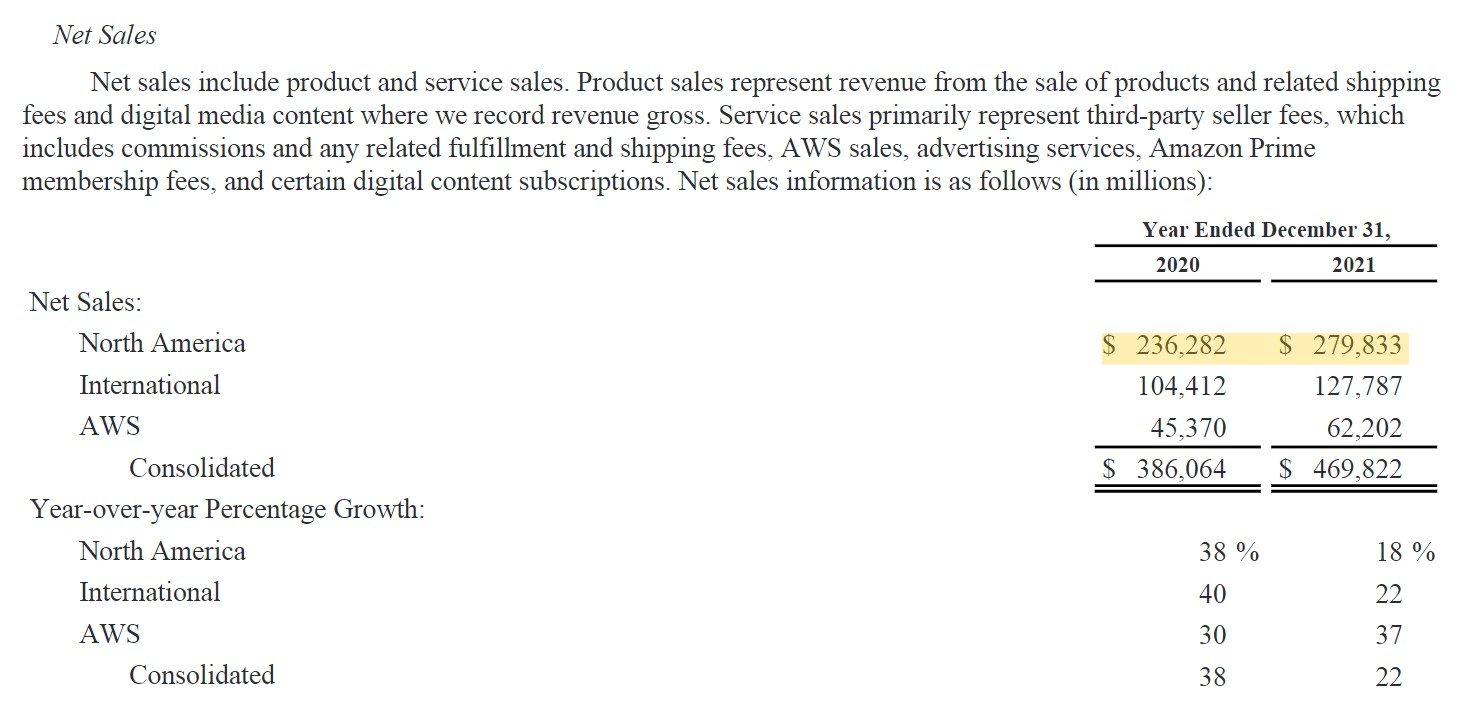Despite the strong growth in the segment and significant contribution towards total revenue in 2021 (60%), the AWS business segment is much more profitable with more favorable unit economics.

The retail side of the business is lower-margin, whereas the revenue from AWS and cloud computing is viewed as higher quality, confirmed by how it contributes close to half of Amazon’s operating income (EBIT).

## Amazon Share Price and 20-for-1 Stock Split

Since the early months of 2022, Amazon’s share price has declined substantially, alongside a general market pullback.

The date on which we’re performing our Amazon valuation analysis is June 14, 2022.

As of the last closing date, Amazon shares were trading for \$102.31 at market close.

• Current Share Price (6/14/22) = \$102.31

The current share price as of the latest close date reflects a ~40% decline since the end of 2021.

Amazon also recently underwent a 20-for-1 stock split that went into effect on June 6, which is the reason it no longer trades in excess of \$2,000 per share.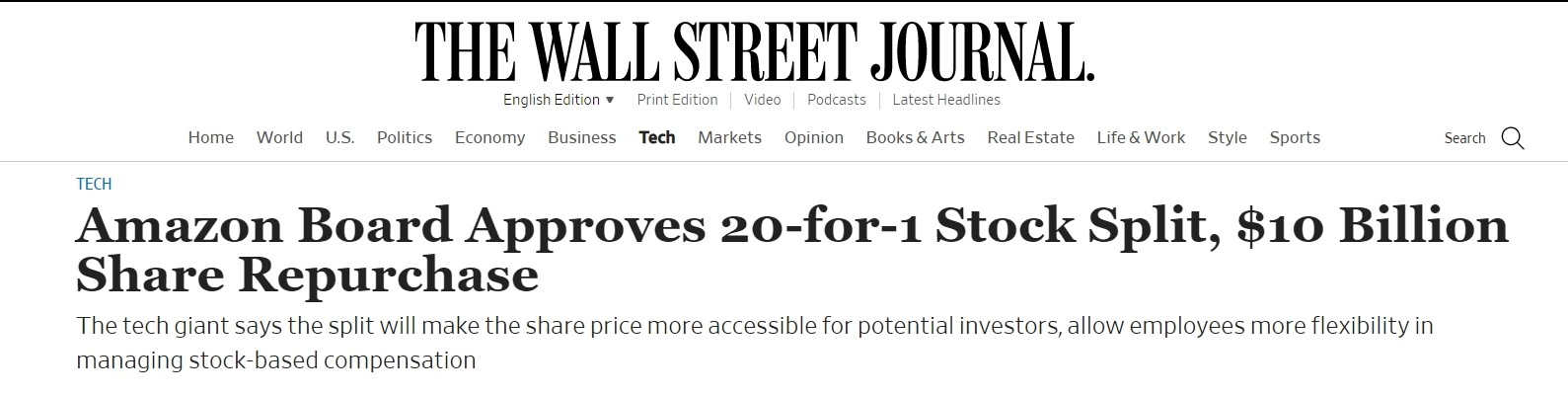Amazon Board Approves 20-for-1 Stock Split (Source: WSJ)

###### Stock Split Impact on Valuation

A stock split refers to a company’s shares being divided into multiple shares, or twenty, in the case of Amazon.

In theory, stock splits should not impact a company’s market capitalization, i.e. its equity value, because they merely cause the number of shares to increase while the ownership stake percentage of each investor remains the same post-split.

However, we’ll soon see over the coming months how well that theory holds up in practice, as the substantially lower share price increases the pool of potential investors that can now afford to purchase a share (and having more buyers generally has a positive correlation with trading prices).

## Amazon Valuation Model – DCF Excel Template

We’ll now move to a modeling exercise, which you can access by filling out the form below.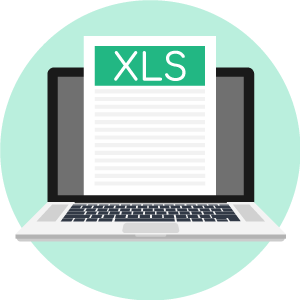Submitting ...

## Historical Income Statement

The first step to building our model is to enter Amazon’s historical financial data into Excel.

While Amazon has actually reported its Q-1 earnings for 2022, our model will only incorporate the high-level takeaways from the 10-Q, rather than creating another column for the performance as of the last twelve months (LTM).

Amazon’s income statement for its latest fiscal year can be found below.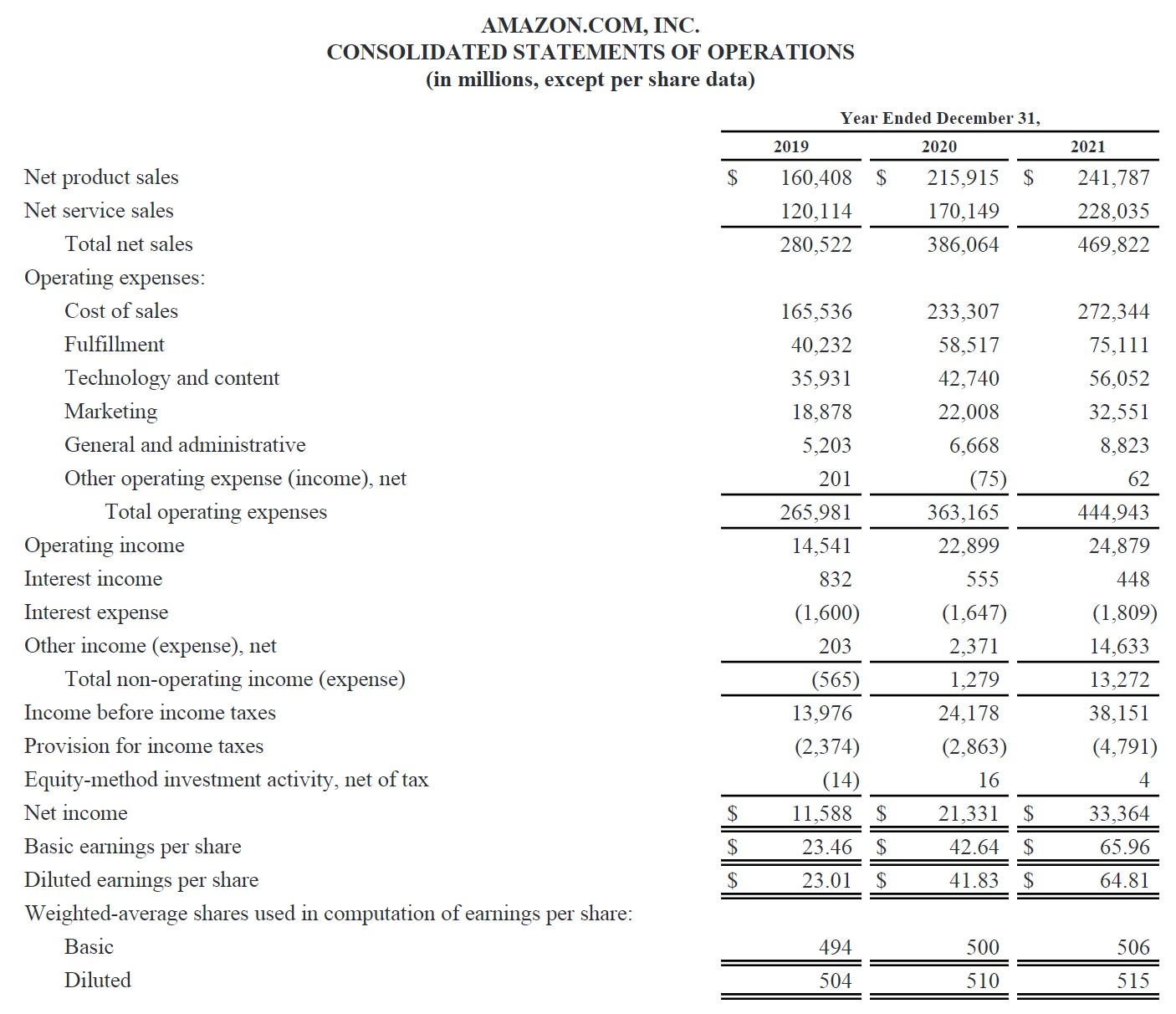Our model will separate the “Cost of Sales” line item from the operating expenses section to calculate gross profit.

In addition, the following four operating expenses will be incorporated into our model:

1. Fulfillment
2. Research and Development
4. Other Operating Expense / (Income), net

“Technology and content” was recorded as R&D while “Marketing” was combined with “General and administrative”.

The completed historical income statement should match the following numbers:

Income Statement
(\$ in millions) 2019A 2020A 2021A
Net Sales \$280,522 \$386,064 \$469,822
(–) Cost of Sales (165,536) (233,307) (272,344)
Gross Profit \$114,986 \$152,757 \$197,478
(–) Fulfillment (40,232) (58,517) (75,111)
(–) Research and Development (35,931) (42,740) (56,052)
(–) Sales, General and Administrative (24,081) (28,676) (41,374)
(–) Other Operating Expense (201) 75 (62)
EBIT \$14,541 \$22,899 \$24,879
(–) Interest Expense (1,600) (1,647) (1,809)
(+) Interest Income 832 555 448
(+) Other Income / (Expense) 203 2,371 14,633
EBT \$13,976 \$24,178 \$38,151
(–) Taxes (2,388) (2,847) (4,787)
Net Income \$11,588 \$21,331 \$33,364

## Non-GAAP Reconciliation

Net income is an accrual-based profit metric (”bottom-line”), and we’ll calculate EBITDA next.

EBITDA reflects a company’s operating profitability and is calculated by subtracting all operating expenses such as COGS, SG&A, and R&D – but not depreciation and amortization (D&A).

By neglecting D&A, EBITDA measures a company’s operating profits without the risk of being distorted by significant non-cash accounting expenses.

The simplest formula for calculating EBITDA is to add D&A to EBIT, but nowadays increasingly more creative adjustments are being made, which must also be taken into account.

In order to keep our model simple, our only two adjustments to EBIT are to add D&A and SBC, which are both non-cash expenses.

1. Depreciation and Amortization: D&A is a non-cash expense that estimates the annual reduction in the value of either fixed assets (PP&E) or intangible assets.
2. Stock-Based Compensation: SBC is a non-cash expense that reduces a company’s taxable income and enables the issuer to compensate (and incentivize) employees while conserving cash – but unlike D&A, SBC represents a real cost incurred to the issuer due to the creation of additional dilution.

It is important to note that stock-based compensation may conserve cash for companies right now but can negatively affect existing and future shareholders through dilution later.

The historical values of D&A and SBC can be found on the cash flow statement.

Operating Assumptions

With our income statement and EBITDA calculation complete, our next step is to calculate the historical margins and ratios that drive our income statement projections.

From 2019 to 2021, an “A” (for “actual”) is placed at the end to indicate those years are historical periods.

On the other hand, the “E” (for “expected”) behind the time period between 2022 and 2026 signifies that those are projected values.

For the historical periods, we’ll calculate our model’s operating assumptions using the following formulas shown below.

###### Operating Assumptions
• Sales Growth Rate = (Current Year / Prior Year) – 1
• Gross Margin = Gross Profit / Sales
• Fulfilment % of Sales = Fulfilment Expense / Sales
• R&D Margin = R&D / Sales
• SG&A Margin = SG&A / Sales
• EBIT Margin = EBIT / Sales
• Effective Tax Rate = Taxes / EBT
• SBC % of Sales = SBC / Sales
• EBITDA Margin = EBITDA / Sales

Based on those three years of historical data and from referencing management guidance (and equity research reports), we’ll use the following forward-looking assumptions.

Operating Assumptions
(\$ in millions) 2019A 2020A 2021A 2022E 2023E 2024E 2025E
Sales Growth Rate NA 37.6% 21.7% 12.5% 18.0% 15.0% 10.0%
Gross Margin 41.0% 39.6% 42.0% 40.0% 41.3% 42.5% 43.8%
Fulfilment % of Sales 14.3% 15.2% 16.0% 16.0% 15.5% 15.0% 14.5%
R&D Margin 12.8% 11.1% 11.9% 12.0% 11.5% 11.0% 10.5%
SG&A Margin 8.6% 7.4% 8.8% 8.5% 8.1% 7.8% 7.4%
EBIT Margin 5.2% 5.9% 5.3% 3.5% 6.1% 8.8% 11.4%
Effective Tax Rate 17.1% 11.8% 12.5% 16.0% 16.0% 16.0% 16.0%
SBC % of Sales 2.4% 2.4% 2.7% 2.5% 2.0% 1.5% 1.0%
EBITDA Margin 15.4% 14.9% 15.3% 12.0% 14.2% 16.2% 18.2%

Note that the EBIT margin and EBITDA margin are NOT projected, hence the font color in our model is black rather than blue to signify that they are calculations rather than hardcodes.

Our revenue assumptions are based on management guidance and the consensus among equity research analysts. Consensus estimates are typically not provided for further than three years into the future.

• Sales Growth Rate Assumptions
• 2022E → 12%
• 2023E → 16.5%
• 2024E → 15.0%
• 2025E → 10.0%
• 2026E → 4.5%

For our remaining operating assumptions – excluding the tax rate which is set at 16.0% and then “straight-lined” across the entire forecast – we’ll make an assumption for what the metric will be for the first year (2022E) and the final year (2026E).

From there, we would then calculate the difference and divide that amount by the number of periods.

The incremental amount is subtracted (or added) to the prior year’s value to come up with a smooth progression.

The assumptions listed in our model can be seen below.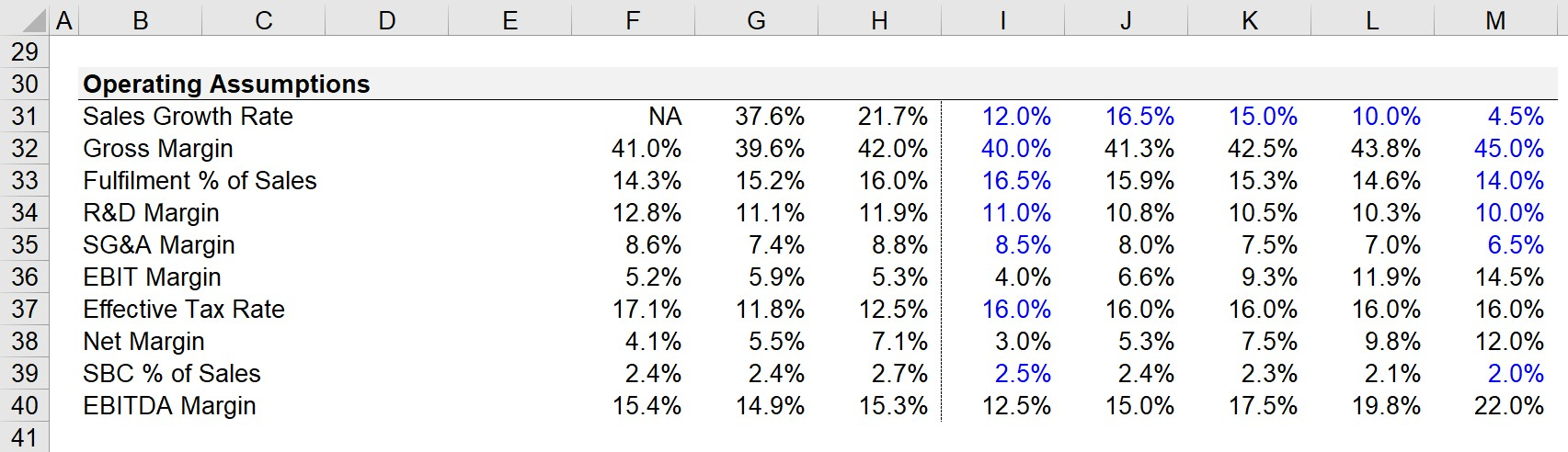Our completed income statement is shown below, however, note that at this point we’ve skipped the interest expense and interest income section since we first need a debt schedule.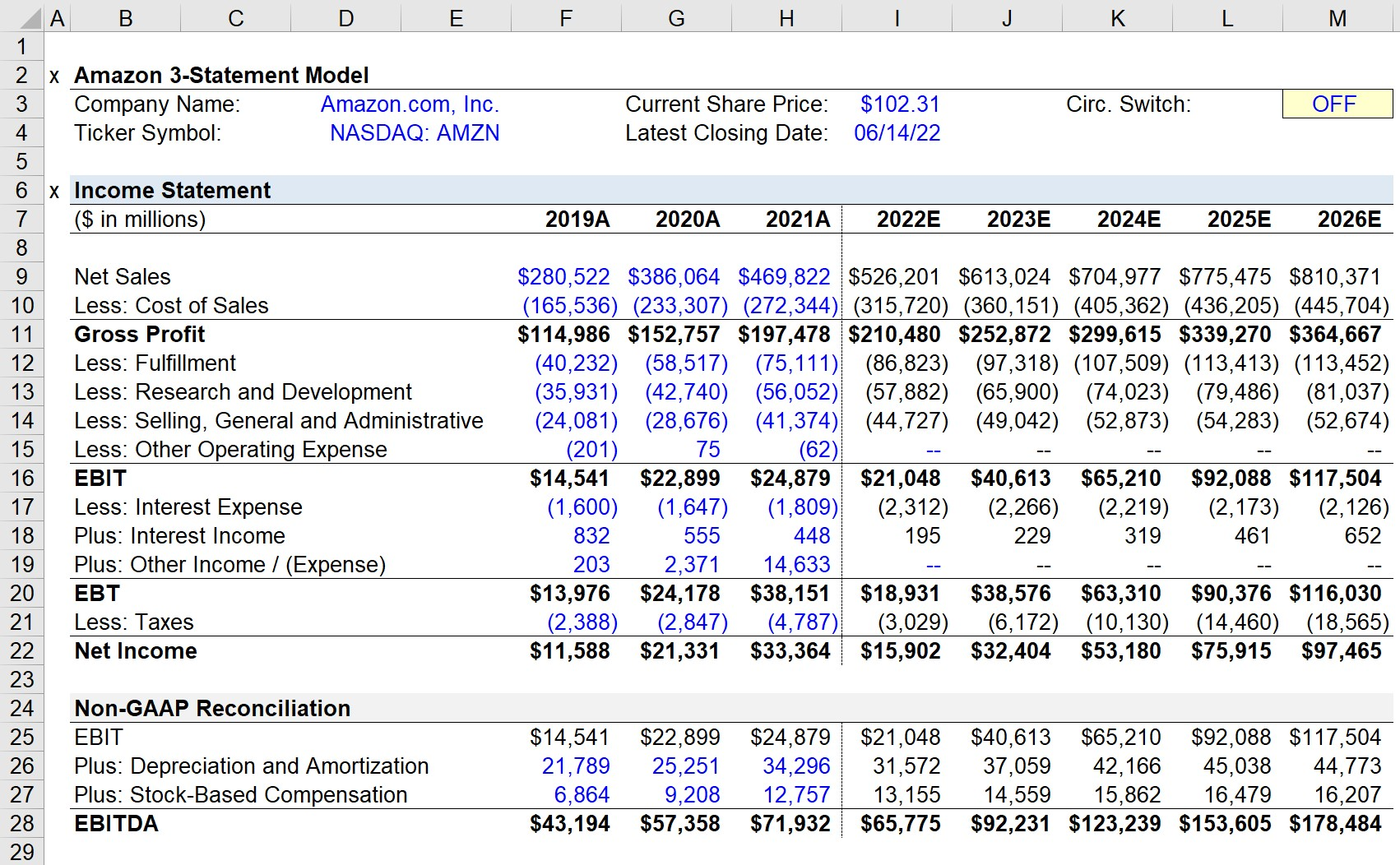## Historical Balance Sheet

The next part is to enter the historical balance sheet data for the years 2020 and 2021.

Unlike the income statement, we’ll only have two years of historical data to reference in the 10-K rather than three.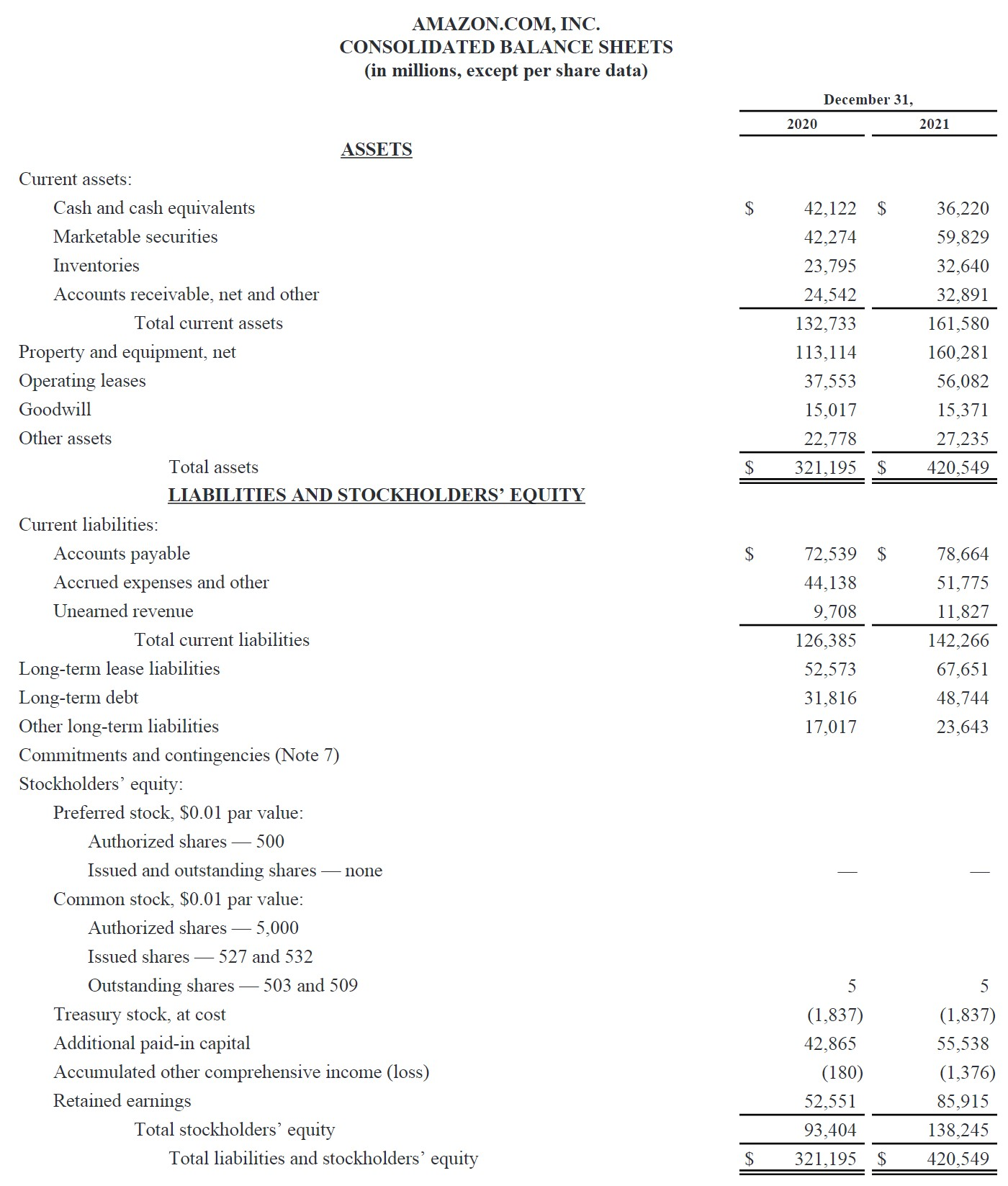The balance sheet typically requires more discretionary adjustments and consolidations of line items that are either insignificant to the final outcome or modeled using the same underlying driver and can thus be analyzed together.

Balance Sheet
(\$ in millions) 2020A 2021A
Cash and Cash Equivalents \$84,396 \$96,049
Inventories 23,795 32,640
Accounts Receivable, net 24,542 32,891
Property, Plant and Equipment, net 113,114 160,281
Goodwill 15,017 15,371
Other Long-Term Assets 60,331 83,317
Total Assets \$321,195 \$420,549
Revolver
Accounts Payable 72,539 78,664
Accrued Expenses 44,138 51,775
Deferred Revenue 9,708 11,827
Long-Term Debt 84,389 116,395
Other Long-Term Liabilities 17,017 23,643
Total Liabilities \$227,791 \$282,304
Common Stock and APIC \$42,870 \$55,543
Treasury Stock (1,837) (1,837)
Other Comprehensive Income / (Loss) (180) (1,376)
Retained Earnings 52,551 85,915
Total Equity \$93,404 \$138,245

Let’s assume that the historical section has been populated, and we’ll now begin to project Amazon’s balance sheet items.

Given our focus on modeling mechanics, as opposed to the granular details of Amazon’s business model, we’ll set the following line items equal to the prior period and then keep them consistent across the entire forecast:

• Goodwill
• Other Long-Term Assets
• Other Long-Term Assets
• Treasury Stock
• Other Comprehensive Income / (Loss)

Starting from 2021A, the value of each line item above remains constant until 2026.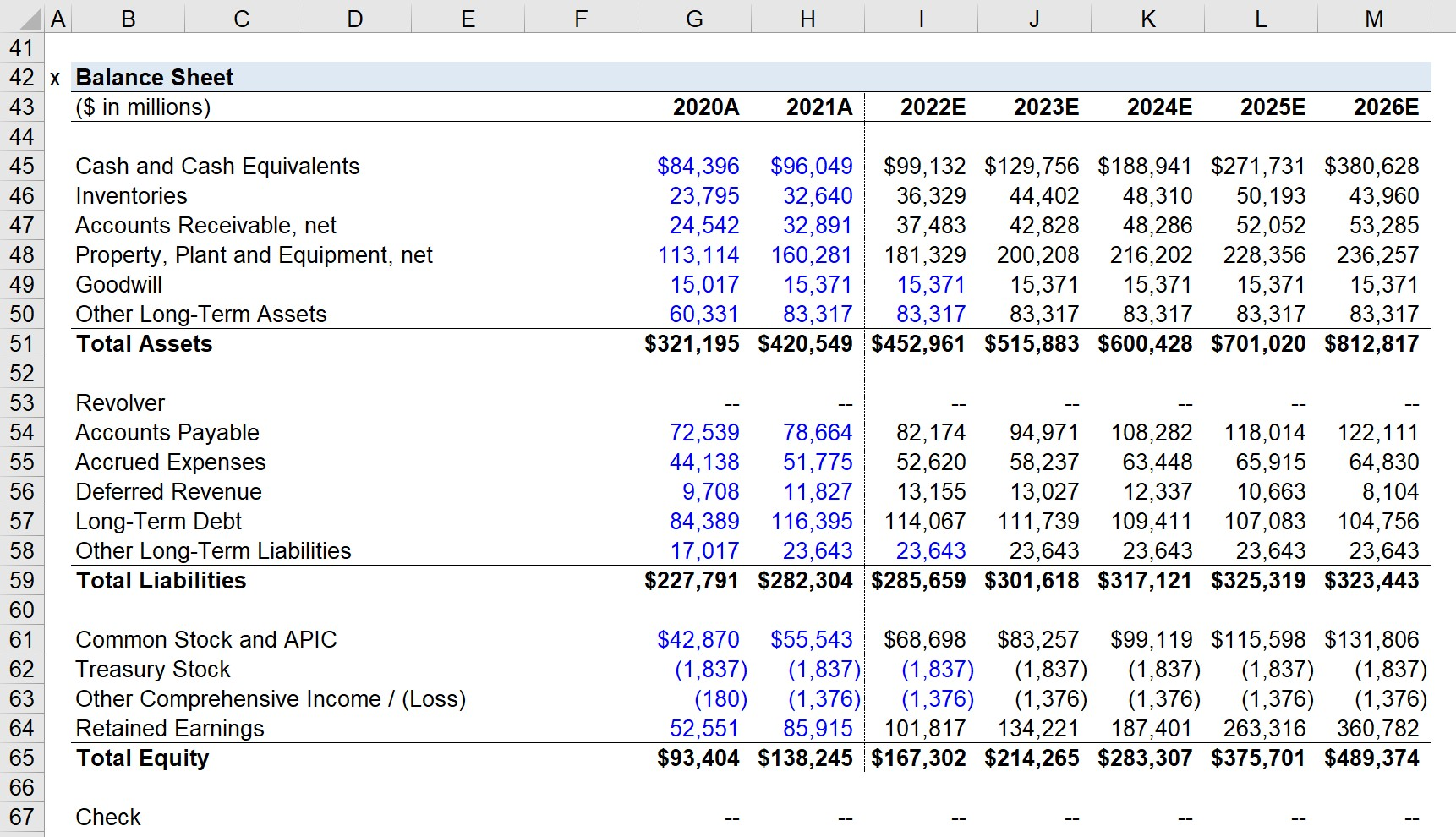## Working Capital Schedule

The first step of projecting the balance sheet is creating the working capital schedule.

There are five operating working capital line items, which will be projected using the following associated metrics:

• Inventory → Inventory Days
• Inventory days are used interchangeably with the term “days inventory outstanding (DIO)” and measure the number of days it takes a company to clear out its inventory.
• Accounts Receivable → A/R Days
• A/R days, also known as “days sales outstanding (DSO)” is the number of days it takes a company to collect cash payments from customers that paid on credit.
• Accounts Payable → A/P Days
• A/P days, or “days payable outstanding (DPO)”, count the number of days that a company can delay paying its suppliers/vendors in cash.
• Accrued Expenses → Accrued Expenses % Revenue
• Accrued expenses can be forecasted as a percentage of SG&A, but in this case, the type of expenses (and size) makes using revenue as the driver more preferable.
• Deferred Revenue → Deferred Revenue % Sales
• Deferred revenue is “unearned” revenue where the cash payment was received before the company provided the product/service to the customer (and in most cases, is projected using sales).

With those historical working capital metrics complete, we’ll repeat the step of setting an assumption for the first and final year and then applying smooth, linear growth from year-to-year.

The formulas for the working capital ratios are as follows:

###### Historical Working Capital Metric Formula
• Inventory Days = (Ending Inventory / Cost of Goods Sold) * 365 Days
• A/R Days = (Ending Accounts Receivable / Revenue) * 365 Days
• A/P Days = (Ending Accounts Payable / Cost of Goods Sold) * 365 Days
• Accrued Expenses % Sales =Accrued Expenses / Sales
• Deferred Revenue % Sales = Deferred Revenue / Sales

Using the above formulas, we can reference the historical numbers to project how the metrics will trend over the next five years.

• Inventory Days
• 2022E = 42 Days
• 2026E = 36 Days
• A/R Days
• 2022E = 26 Days
• 2026E = 24 Days
• A/P Days
• 2022E = 95 Days
• 2026E = 100 Days
• Accrued Expenses % Sales
• 2022E = 10.0%
• 2026E = 8.0%
• Deferred Revenue % Sales
• 2022E = 2.5%
• 2026E = 1.0%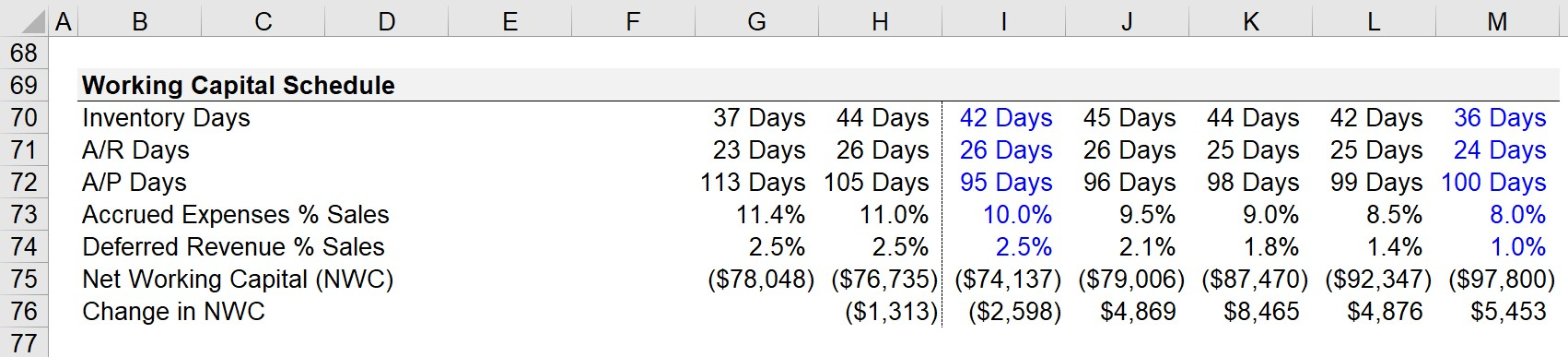Once the assumptions are all entered, the next step is to project the corresponding metric on the balance sheet using the formulas below.

###### Working Capital Forecasting Formula
• Inventories = – Inventory Days * Cost of Sales / 365
• Accounts Receivable = A/R Days * Revenue / 365
• Accounts Payable = – A/P Days * COGS / 365
• Accrued Expense = (Accrued Expense % Revenue) * Revenue
• Deferred Revenue = (Deferred Revenue % Revenue) * Revenue

At this point, our working capital line items are complete, and we can calculate the “Net Working Capital (NWC)” metric by subtracting the sum of operating current assets by the sum of operating current liabilities.

In order to see the net cash impact from operations, we must calculate the change in NWC by subtracting the current year’s NWC from the prior year’s NWC.

###### Net Working Capital (NWC) Metrics
• Net Working Capital (NWC) = (Inventory + Accounts Receivable) – (Accounts Payable + Accrued Expense + Deferred Revenue)
• Change in NWC = Prior Year NWC – Current Year NWC

If the change in NWC is positive, the impact on cash is negative (i.e. a “cash outflow”), whereas a negative change in NWC causes cash to increase (a “cash inflow”).

## PP&E Schedule

We’ll now move on to forecasting “Property, Plant & Equipment”, which refers to the long-term fixed assets belonging to a company.

Unlike current assets, PP&E is expected to provide positive monetary benefits to the company for more than one year, i.e. the useful life assumption exceeds twelve months.

The carrying value of PP&E on the balance sheet is affected primarily by two line items: capital expenditures (i.e. the purchase of PP&E) and depreciation (i.e. the allocation of capex across the useful life of the asset).

###### PP&E Roll-Forward Formula
• Ending PP&E = Beginning PP&E + Capex – Depreciation

To forecast PP&E, we must first project capex and depreciation.

• Capex is most often projected as a percentage of revenue, with management guidance also taken into consideration.
• Depreciation can also be explicitly provided by management, but most models will project it as a percentage of either capex or revenue.

Once companies mature, capex as a percentage of revenue tends to decline as opportunities for growth investments gradually decline (and the ratio between depreciation-to-capex converges over time to 1.0x, or 100%).

Our model assumes that capex is 10% of revenue in 2022 but decreases to 6.5% of revenue by the end of 2026.

During the same time frame, D&A is assumed to be 60% of capex in 2022 but climbs to 85% of capex by the end of 2026.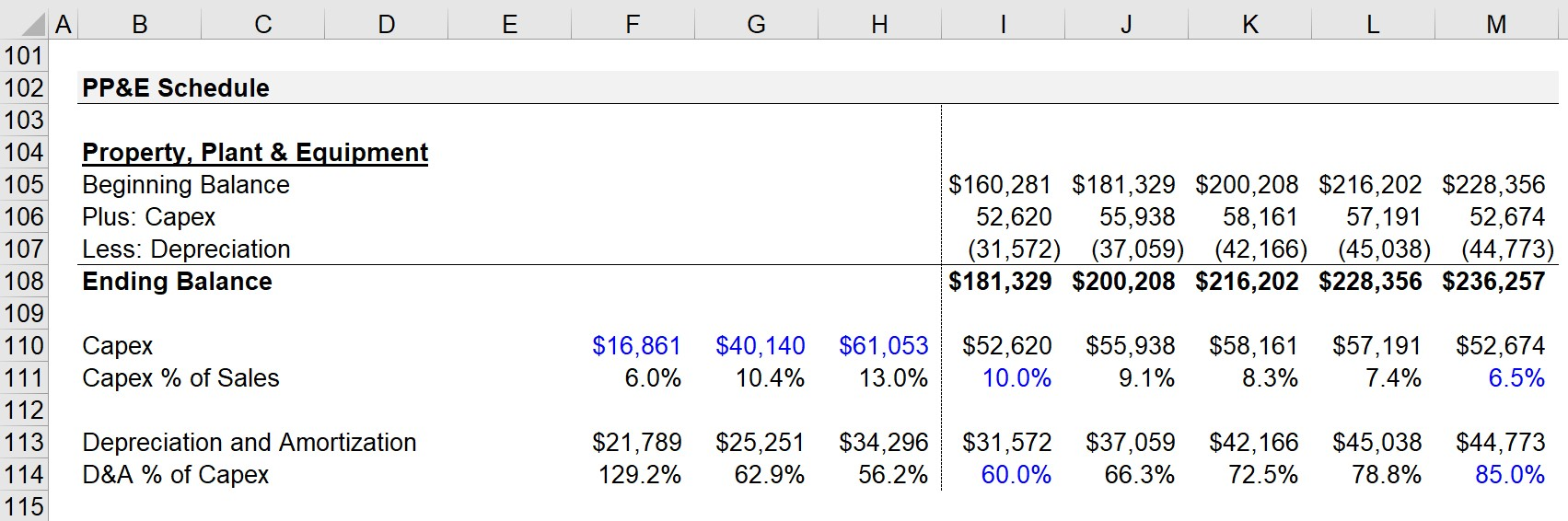## Equity Section

The assets and liabilities section of our model is now finished except for the debt-related items, which we’ll return to later after the debt schedule is done.

The four-line items of our equity section are calculated using the following formulas:

1. Common Stock and APIC = Prior Balance + Stock-Based Compensation
2. Treasury Stock = Prior Balance – Share Buybacks
3. Other Comprehensive Income / (Loss) = Straight-Line
4. Retained Earnings = Prior Balance + Net Income – Dividends

## Cash Flow Statement (CFS)

Unlike the other two financial statements, the historical cash flow statement does not need to be entered into our model.

However, there are certain historical line items that we must reference, such as:

• Depreciation and Amortization (D&A)
• Stock-Based Compensation
• Capital Expenditures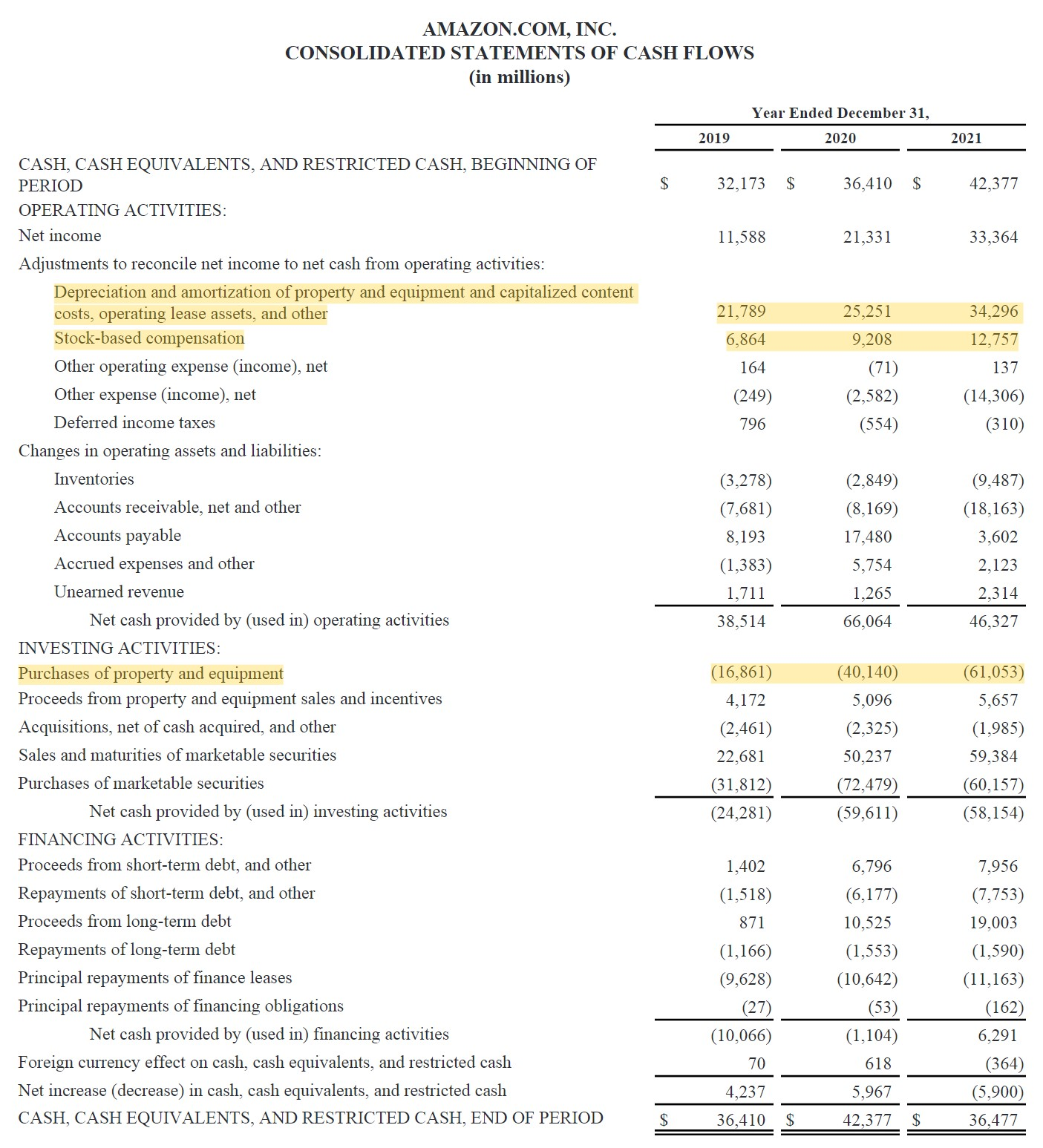The starting line item of the CFS is net income, which flows in from the bottom of the income statement.

Net income is an accrual-based profit metric, so we must adjust it by adding back any non-cash line items such as D&A and stock-based compensation, where there was no actual cash outflow.

Once the non-cash line items are added back, the changes in working capital must be incorporated.

The guidelines for changes in working capital and how they affect cash flows are as follows:

• Increase in NWC → Decrease in Cash Flows (“Use”)
• Decrease in NWC → Increase in Cash Flows (“Source”)

The first section of our CFS, the cash flow from operating activities, is now done, so we can move to the cash flow from investing activities.

The primary line item to pay attention to is capital expenditures, namely because it is recurring and a core part of a company’s operations.

Capex spend declining over time typically means that a company is running out of growth opportunities.

Based on Amazon’s capex spending in 2020, the pandemic was a tailwind for companies such as Amazon – yet the company had to reinvest significant amounts of capital to fulfill the overwhelming demand from consumers.We’re now at the third and final section of our cash flow statement, but since all three line items are related to financing, we can skip this entire section and return to it once the debt schedule is complete.

However, we can still create the cash roll-forward schedule, in which the ending cash balance is equal to the beginning cash balance plus the net change in cash, which is the sum of all three CFS sections.

###### Cash Roll-Forward Formula
• Ending Cash Balance = Beginning Cash Balance + Net Change in Cash

Where:

• Net Change in Cash = CFO + CFI + CFF

## Debt Schedule

We’re now close to being done with our 3-statement model for Amazon, and all that remains is the debt schedule (and the parts that we skipped earlier).

The first step is to calculate the amount of cash available to service debt.

• Cash Available for Revolver = Beginning Cash Balance + Excess Free Cash Flow (FCF) – Minimum Cash Balance

The result will reflect whether Amazon needs to draw from the revolver (i.e. insufficient funds) or if it can afford to pay down its outstanding revolver balance.

Our model’s debt schedule is comprised of two debt securities:

Amazon currently has two revolving credit facilities in place:

1. Secured Revolver
2. Unsecured Revolver
• Credit Facility Size = \$7 billion
• Commitment Fee = 0.04%

For both facilities, we’ll assume an interest rate of 2.0%, which will be multiplied by the average of the beginning and ending debt balance for each period.

• Revolver Interest Rate = 2.0%
• Revolver Interest Expense = Average Revolver Balance * Interest Rate
###### Circularity Switch

Since forecasting interest expense introduces a circularity into our model, we’ll create a circularity switch and name the cell “Circ”.

From that point onward, all interest expense and interest income formulas will contain the “IF” statement in front, where if the “Circ” cell is set to “0” (i.e. the circuit breaker is turned on), a value of the output is set to “0” as well to prevent errors from popping up in our model.

The mechanics of our revolvers will be set up so that the unsecured revolver will only be drawn from if the secured revolver is fully drawn down.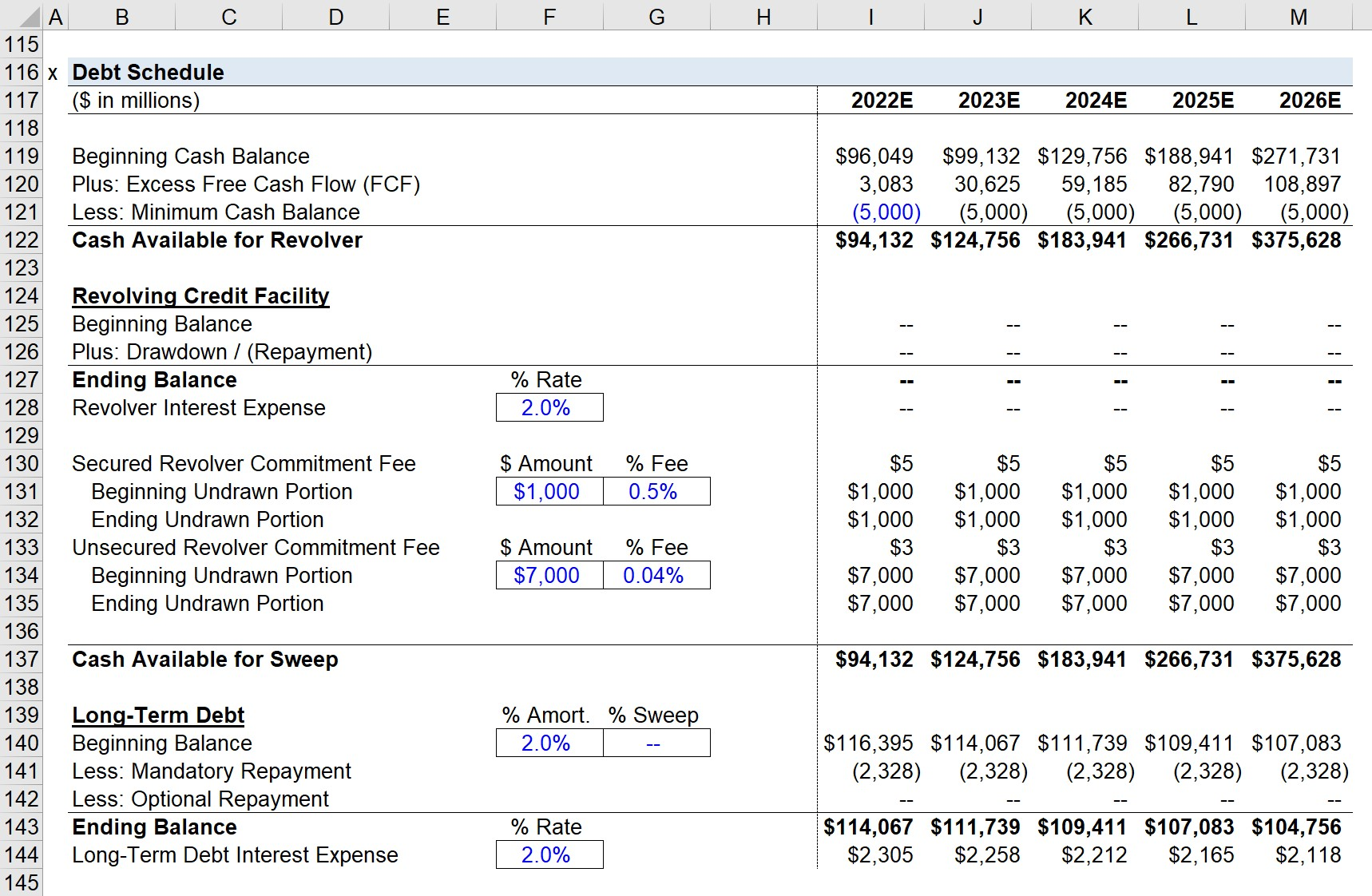While the revolver, in this case, is drawn from (or paid down) as-needed, long-term debt can also come with mandatory repayments of the principal throughout the borrowing term.

Here, we’ll assume the annual principal amortization is 2.0%, which appears to be in line with Amazon’s historical repayments of debt.

The mandatory repayment formula will multiply the % amortization assumption by the original principal amount, with a “MIN” function to ensure the balance of the debt does not decline below zero.

We also added an optional repayment line, where Amazon can repay the debt principal earlier than scheduled if it desires to, but our model has this set to zero.

To ensure that there are no linking mistakes, we can create a separate interest schedule to calculate our total interest expense and net interest expense.

The ending balance for long-term debt is equal to the beginning balance adjusted for any new issuances of debt, mandatory repayment, and optional repayments.

###### Long-Term Debt Formula
• Long-Term Debt Ending Balance = Beginning Balance + New Debt Issuances – Mandatory Repayment

The total interest expense and interest income are both linked back to the income statement, but be sure to make sure the sign conventions are correct.

• Interest Expense → Negative (–)
• Interest Income → Positive (+)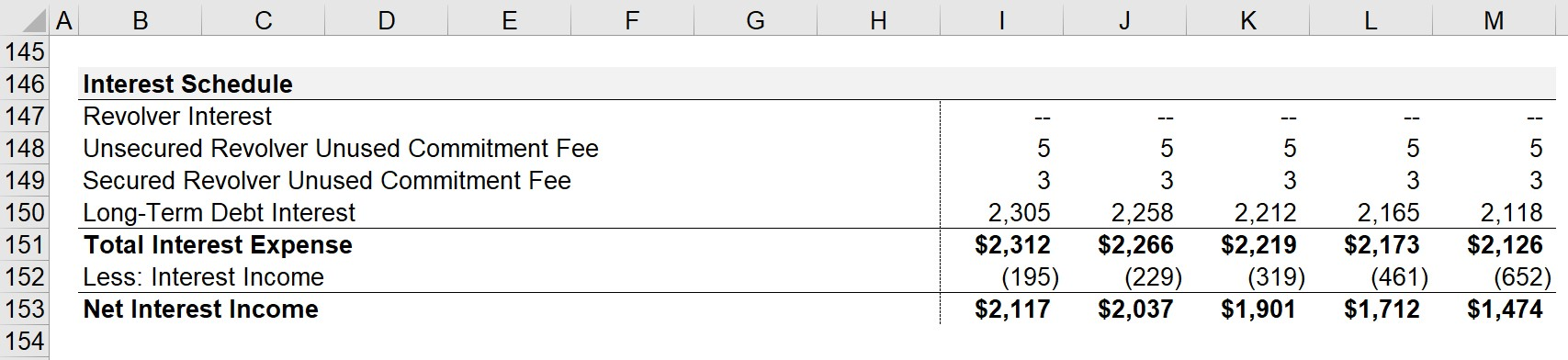The final step in our 3-statement model is then to link our ending debt balances to our balance sheet.

If done correctly, our balance sheet should now properly balance, i.e. assets = liabilities + equity.

## Free Cash Flow Build (FCF)

We’re now ready to create a new tab for our DCF model, where we’ll calculate Amazon’s free cash flow to firm (FCFF).

The first step is to link towards EBITDA from our financial statement model and then subtract D&A to calculate EBIT for each projected year.

We will then tax-affect EBIT to arrive at Amazon’s net operating profit after taxes (NOPAT), which is the starting point for our FCFF formula.

###### Free Cash Flow to Firm (FCFF) Formula
• FCFF = NOPAT + D&A – Change in NWC– Capex

In the prior step, we can simply link to D&A from above, whereas the change in NWC and Capex can be linked from our cash flow statement.

To ensure the sign convention is correct, it is recommended to link directly to the cash flow statement, rather than our schedules.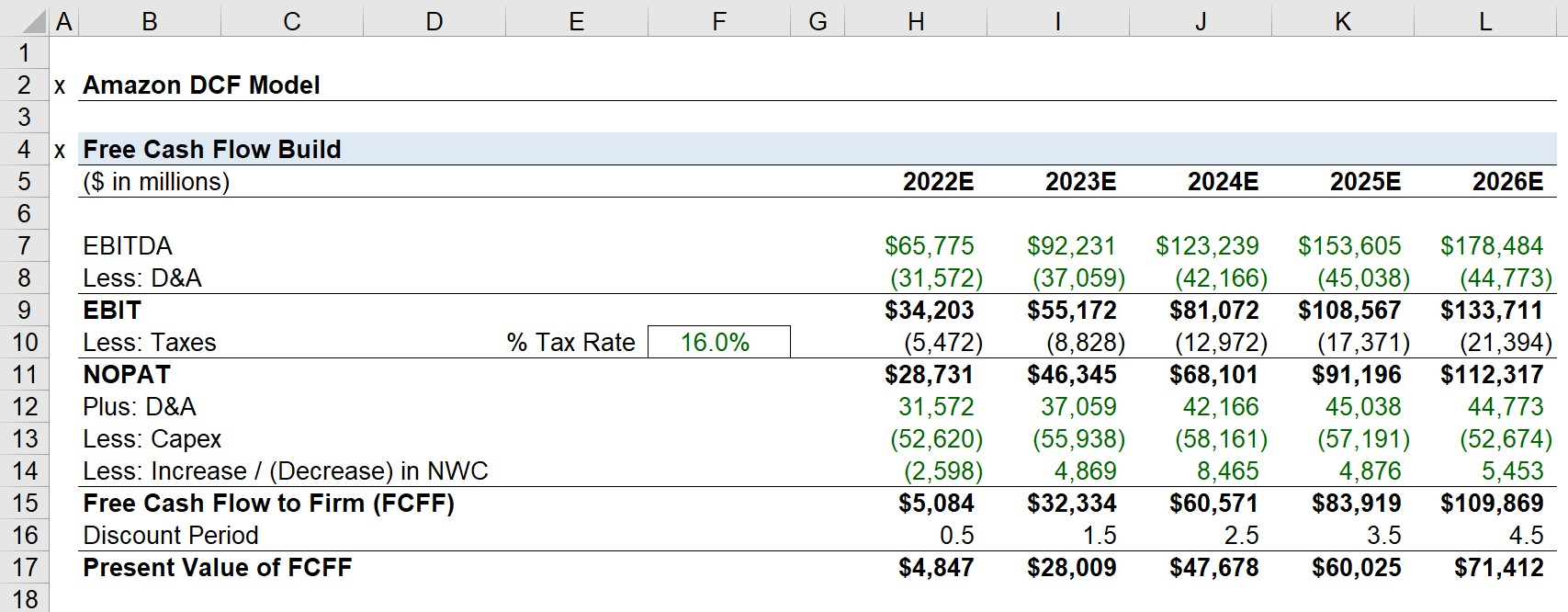Once the FCFF for the five-year forecast period is calculated, we’ll list the discount factor in the line underneath it.

The discount factor formula uses the “COUNTA” Excel function to count the number of years that have passed and subtracts 0.5 from it to abide by the mid-year convention.

Each FCFF can then be discounted to the present date by dividing the FCFF amount by (1 + WACC) raised to the discount factor.

But since we have not yet calculated the WACC, we’ll pause for now.

## WACC Calculation

The weighted average cost of capital (WACC) is the discount rate used for an unlevered DCF.

WACC represents the opportunity cost of an investment based on other investments with comparable risk profiles.

The WACC formula multiplies the equity weight (% of capital structure) by the cost of equity and adds it to the debt weight (% of capital structure) multiplied by the tax-affected cost of debt.

###### WACC Formula
• WACC = [ke * (E / (D + E)] + [kd * (D / (D + E)]

Where:

• E / (D + E) → Equity Weight (%)
• D / (D + E) →  Debt Weight (%)
• ke → Cost of Equity
• kd → After-Tax Cost of Debt

The pre-tax cost of debt can be calculated by dividing Amazon’s total interest expense by the total debt outstanding on its balance sheet.

However, the cost of debt must be tax-affected since interest expense is tax-deductible, i.e. the interest “tax shield”, unlike the dividends issued to shareholders.

###### Cost of Debt Formula
• Pre-Tax Cost of Debt = Interest Expense / Total Debt Outstanding
• After-Tax Cost of Debt = Pre-Tax Cost of Debt * (1 – Tax Rate %)

Calculating the cost of debt (kd) is relatively straightforward since bank loans and corporate bonds possess readily observable interest rates on sources such as Bloomberg.

The cost of debt represents the minimum return that debt holders (i.e. lenders) require before bearing the burden of lending capital to a particular borrower.

We’ll start by calculating Amazon’s pre-tax cost of debt.

• Pre-Tax Cost of Debt = \$1.81 billion / \$116.4 billion = 1.6%

Next, we must tax-affect the rate since interest is tax-deductible.

While the effective tax rate from 2021 could be used here, we’ll use our normalized tax rate assumption of 16.0% instead.

• After-Tax Cost of Debt = 1.6% * (1 – 16%) = 1.3%

The cost of equity is calculated using the capital asset pricing model (CAPM), which states that the expected return is a function of a company’s sensitivity to the broader market, most often the S&P 500 index.

###### CAPM Formula
• Cost of Equity (Re) = Risk-Free Rate + Beta × Equity Risk Premium

There are three inputs to the CAPM formula:

1. Risk-Free Rate (rf): The risk-free rate is the rate of return received on default-free investments, which serves as the minimum return hurdle for riskier assets. In theory, the risk-free rate should reflect the yield to maturity (YTM) on government-issued bonds of equal maturity as the time horizon of projected cash flows (i.e. default-free issuances).
2. Equity Risk Premium (ERP): The ERP is the incremental risk from investing in the equities market rather than risk-free securities such as government-issued bonds. Thus, ERP is the excess return above the risk-free rate and has historically ranged from around 4% to 6%. Formulaically, the ERP equals the difference between the 1) expected market return and 2) the risk-free rate.
3. Beta (β): Beta is a measure of risk that measures the sensitivity of individual securities relative to the broader market, i.e. the systematic risk, which is the non-diversifiable risk component that cannot be eliminated from diversification.

The next step is to calculate the cost of equity using the capital asset pricing model (CAPM).

The yield on 10-year U.S. government bonds is approximately 3.4%, which we will use as our risk-free rate.

According to Capital IQ, Amazon’s beta is 1.24, and the recommended equity risk premium (ERP) by Duff & Phelps is 5.5%, so we now have all the necessary inputs.

• Cost of Equity (ke) = 3.4% + (1.24 * 5.5%)
• ke = 10.2%

The final step before we calculate our WACC is to determine the capital structure weights of debt and equity.

While technically the market value of debt should be used, the market value of debt rarely deviates very far from the book value, particularly for companies like Amazon.

Further, we’ll also be using net debt rather than total gross debt, since the cash sitting on the balance sheet could be used to pay down a portion (or all) of the outstanding debt.

Amazon’s equity value on the date of our valuation is \$1.041 trillion, so we’ll add that to net debt to calculate the percentage contribution of each capital source.

• Net Debt = 1.9% of Total Capitalization
• Equity Value = 98.1% of Total Capitalization

Our WACC can now be calculated using the formula below, which comes out to 10.0%.

• WACC = (1.3% * 1.9%) + (10.2% * 98.1%) = 10.0%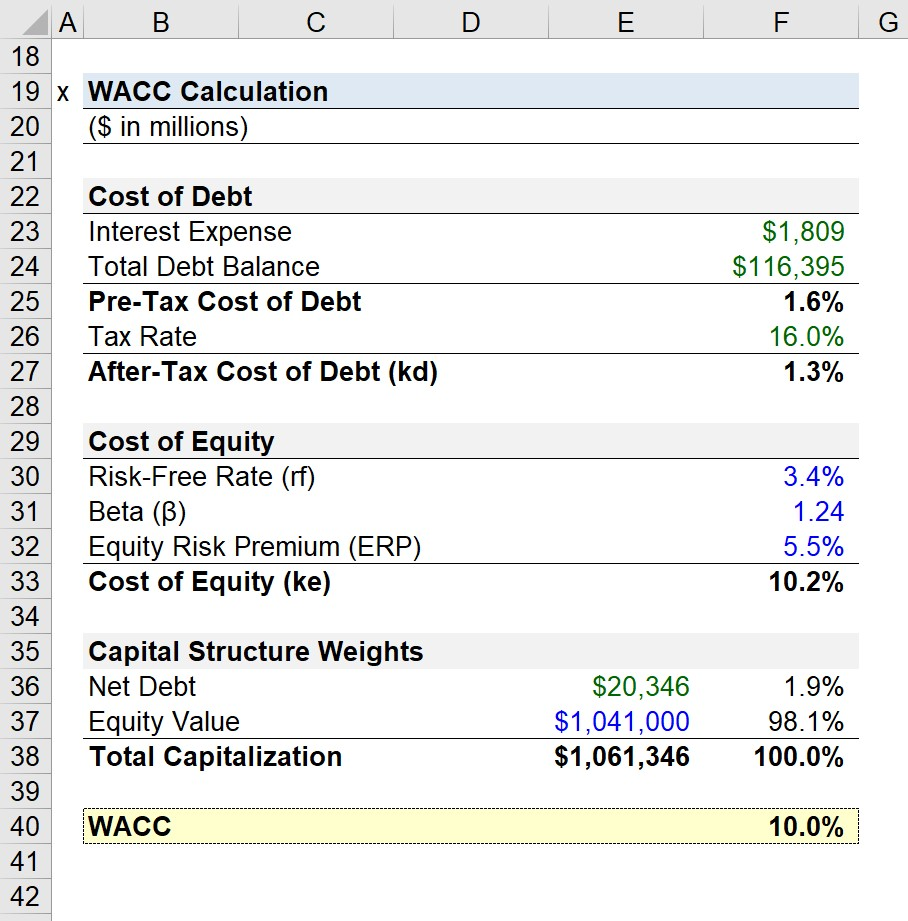## Present Value of Free Cash Flows (FCFs)

Since we now have the WACC, the FCFFs we projected earlier can be discounted to the current date.

###### PV of Free Cash Flow to Firm Formula
• PV of FCFF = FCFF / (1 + WACC)^Discount Factor

The sum of the present value (PV) of those cash flows equals approximately \$212 billion.

• 2022E = \$5,084 million / (1+10.0%)^0.5
• PV of FCFF = \$4,847 million
• 2023E = \$32,334 million / (1+10.0%)^1.5
• PV of FCFF = \$47,678 million
• 2024E = \$60,571 million / (1+10.0%)^2.5
• PV of FCFF = \$47,678 million
• 2025E = \$83,919 million/ (1+10.0%)^3.5
• PV of FCFF = \$60,025 million
• 2026E = \$109,869 million / (1+10.0%)^4.5
• PV of FCFF = \$71,412 million

## Terminal Value Calculation – Perpetuity Growth Approach

We’ll use the perpetuity growth approach to estimate the terminal value, with a long-term growth rate assumption of 3.0%.

The FCF in the final year of the explicit forecast period will be grown by 3.0% into perpetuity.

• Final Year FCF x (1 + g) = \$113.2 billion

In order to calculate the terminal value as of the final year, we’ll divide the \$113.2 billion by the WACC net of the long-term growth rate.

• Terminal Value in Final Year = \$113.2 billion / (10.0% – 3.0%) = \$1,605.8 trillion

However, since that resulting value represents the terminal value as of the final year of our forecast period, we must then discount it to the present day using the same methodology as we used for the Stage 1 FCFFs.

• Present Value of Terminal Value (Stage 2) =\$1,605.8 trillion / (1+10.0%)^(4.5)
• PV of Terminal Value = \$1,043.8 trillion

## Amazon DCF Valuation – Implied Share Price

Upon adding the Stage 1 and Stage 2 values, the total enterprise value (TEV) comes out to \$1.26 trillion.

• Total Enterprise Value (TEV) = \$211,971 million + \$1,043,749 million
• TEV = \$1.26 trillion

In order to calculate equity value, we must then subtract net debt, i.e. subtract total debt and add cash and cash equivalents.

• Equity Value = \$1.26 trillion – \$116,395 billion + \$96,049 billion
• Equity Value = \$1.24 trillion

The final step that precedes our share price calculation is figuring out the total number of diluted shares outstanding.

For the sake of time, we’ll skip that tedious process by just taking the pre-split diluted shares outstanding (i.e. common shares + stock-based awards outstanding) of 523 million.

Amazon underwent a 1-for-20 stock-split ratio, as mentioned earlier, so we’ll simply adjust the diluted share count by multiplying 523 million shares by 20.

• Split-Adjusted Diluted Share Count = 10.46 billion

We can then determine Amazon’s implied share price by dividing its equity value by our estimate of its total diluted shares outstanding.

• Implied Share Price = \$1.24 trillion / 10,460 million = \$118.10

Compared to the current share price of \$102.31, the potential upside according to our model is 15.4%, i.e. Amazon’s shares are currently mispriced by the market and are trading at a discount.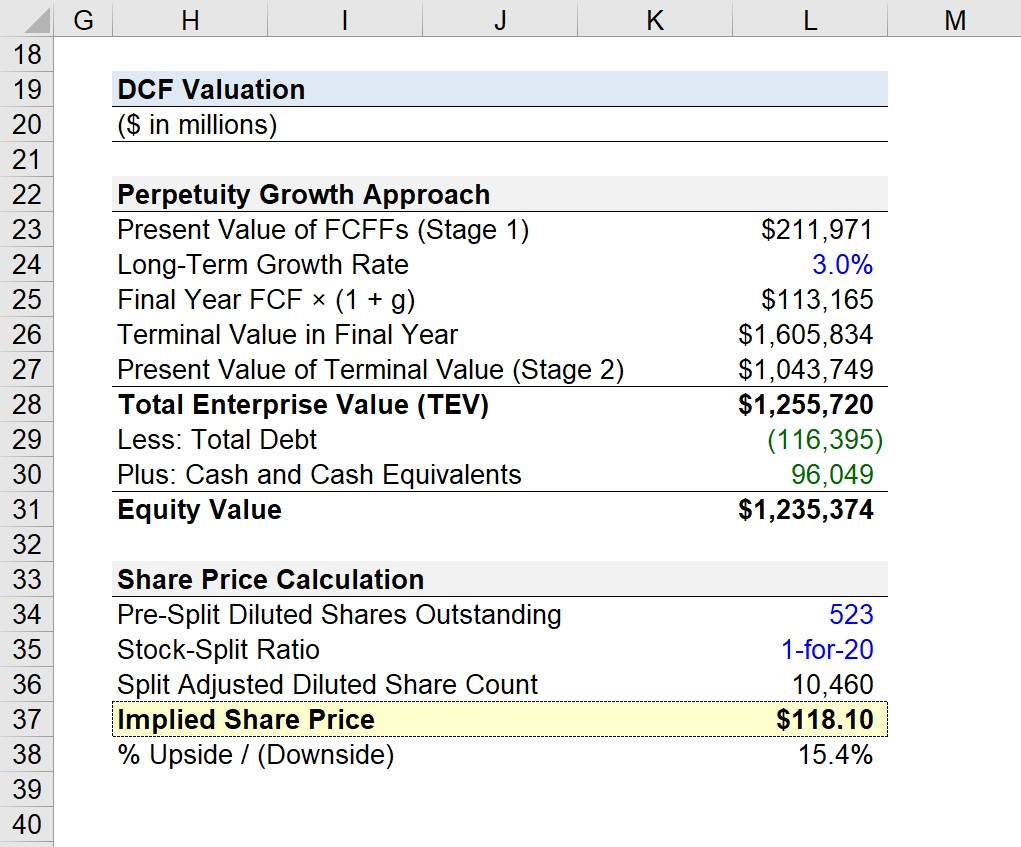## Amazon DCF Valuation Model Video Tutorial

For those who prefer video over text, the following two videos show Ben – a former investment banking analyst at JP Morgan – building a DCF valuation for Amazon from scratch.

However, please note that the DCF model we built here is different from the one Ben built.

Despite the differences in model assumptions and structure, the core theory behind the DCF analysis still remains conceptually the same.

Just as a quick disclaimer, Wall Street Prep is the proud sponsor of rareliquid’s Amazon DCF video.

So if you’re interested in purchasing any one of our course offerings – while also supporting the rareliquid YouTube channel – enter the code “RARELIQUID” to receive 20% off.Step-by-Step Online Course

#### Everything You Need To Master Financial Modeling

Enroll in The Premium Package: Learn Financial Statement Modeling, DCF, M&A, LBO and Comps. The same training program used at top investment banks.Inline FeedbacksLearn Financial Modeling Online

Everything you need to master financial and valuation modeling: 3-Statement Modeling, DCF, Comps, M&A and LBO.

X

The Wall Street Prep Quicklesson Series

7 Free Financial Modeling Lessons

Get instant access to video lessons taught by experienced investment bankers. Learn financial statement modeling, DCF, M&A, LBO, Comps and Excel shortcuts.